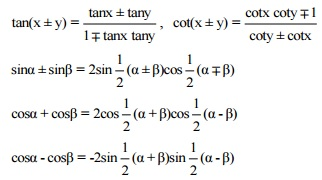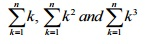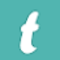### Course Structure

 Unit Topic Chapters of NCERT Marks I. Sets and Functions Chapter 1, 2, 3 29 II. Algebra Chapter 4, 5, 6, 7, 8, 9 37 III. Co-ordinate Geometry Chapter 10, 11, 12 13 IV. Calculus Chapter 13 6 V. Mathematical Reasoning Chapter 14 3 VI. Statistics and Probability Chapter 15, 16 12 Total 100

### Unit-I: Sets and Functions

1. Sets

Sets and their representations.Empty set.Finite and Infinite sets.Equal sets.Subsets.Subsets of a set of real numbers especially intervals (with notations). Power set. Universal set. Venn diagrams. Union and Intersection of sets.Difference of sets. Complement of a set. Properties of Complement Sets.

2. Relations & Functions

Ordered pairs, Cartesian product of sets. Number of elements in the cartesian product of two finite sets. Cartesian product of the sets of real (upto R x R). Definition of relation, pictorial diagrams, domain, co-domain and range of a relation. Function as a special kind of relation from one set to another. Pictorial representation of a function, domain, co-domain and range of a function. Real valued functions, domain and range of these functions: constant, identity, polynomial, rational, modulus, signum, exponential, logarithmic and greatest integer functions, with their graphs. Sum, difference, product and quotients of functions.

3. Trigonometric Functions

Positive and negative angles. Measuring angles in radians and in degrees and conversion from one measure to another.Definition of trigonometric functions with the help of unit circle. Truth of the identity sin2 x+cos2 x=1, for all x. Signs of trigonometric functions. Domain and range of trignometric functions and their graphs. Expressing sin (x±y) and cos (x±y) in terms of sinx, siny, cosx  & cosy and their simple applications. Deducing the identities like the following:Identities related to sin 2x, cos2x, tan 2x, sin3x, cos3x and tan3x. General solution of trigonometric equations of the type sin y = sin a, cos y = cos a and tan y = tan a.

### Unit-II: Algebra

1. Principle of Mathematical Induction

Process of the proof by induction, motivating the application of the method by looking at natural numbers as the least inductive subset of real numbers. The principle of mathematical induction and simple applications.

2. Complex Numbers and Quadratic Equations

Need for complex numbers, especially √−1, to be motivated by inability to solve some of the quardratic equations. Algebraic properties of complex numbers.Argand plane and polar representation of complex numbers. Statement of Fundamental Theorem of Algebra, solution of quadratic equations (with real coefficients) in the complex number system. Square root of a complex number.

3. Linear Inequalities

Linear inequalities. Algebraic solutions of linear inequalities in one variable and their representation on the number line.Graphical solutions of linear inequalities in two variables.Graphical method of finding a solution of system of linear inequalities in two variables.

4. Permutations and Combinations

Fundamental principle of counting. Factorial n. (n!) Permutations and combinations, derivation of formulae for 𝑛𝑃𝑟 and 𝑛𝐶𝑟 and their connections, simple applications.

5. Binomial Theorem

History, statement and proof of the binomial theorem for positive integral indices.Pascal's triangle, General and middle term in binomial expansion, simple applications.

6. Sequence and Series

Sequence and Series. Arithmetic Progression (A. P.). Arithmetic Mean (A.M.) Geometric Progression (G.P.), general term of a G.P., sum of first n terms of a G.P., infinite G.P. and its sum, geometric mean (G.M.), relation between A.M. and G.M. Formulae for the following special sums### Unit-III: Coordinate Geometry

1. Straight Lines

Brief recall of two-dimensional geometry from earlier classes. Shifting of origin. Slope of a line and angle between two lines. Various forms of equations of a line: parallel to axis, point-slope form, slope intercept form, two-point form, intercept form and normal form. General equation of a line.Equation of family of lines passing through the point of intersection of two lines. Distance of a point from a line.

2. Conic Sections

Sections of a cone: circle, ellipse, parabola, hyperbola, a point, a straight line and a pair of intersecting lines as a degenerated case of a conic section. Standard equations and simple properties of parabola, ellipse and hyperbola.Standard equation of a circle.

3. Introduction to Three–dimensional Geometry

Coordinate axes and coordinate planes in three dimensions. Coordinates of a point. Distance between two points and section formula.

### Unit-IV: Calculus

1. Limits and Derivatives

Derivative introduced as rate of change both as that of distance function and geometrically.

Intuitive idea of limit. Limits of polynomials and rational functions trigonometric, exponential and logarithmic functions. Definition of derivative relate it to scope of tangent of the curve, Derivative of sum, difference, product and quotient of functions. Derivatives of polynomial and trigonometric functions.

### Unit-V: Mathematical Reasoning

1. Mathematical Reasoning

Mathematically acceptable statements. Connecting words/ phrases - consolidating the understanding of "if and only if (necessary and sufficient) condition", "implies", "and/or", "implied by", "and", "or", "there exists" and their use through variety of examples related to real life and Mathematics. Validating the statements involving the connecting words, Difference between contradiction, converse and contrapositive.

### Unit-VI: Statistics and Probability

1. Statistics

Measures of dispersion: Range, mean deviation, variance and standard deviation of ungrouped/grouped data. Analysis of frequency distributions with equal means but different variances.

2. Probability

Random experiments; outcomes, sample spaces (set representation). Events; occurrence of events, 'not', 'and' and 'or' events, exhaustive events, mutually exclusive events, Axiomatic (set theoretic) probability, connections with other theories studied in earlier classes. Probability of an event, probability of 'not', 'and' and 'or' events.

#### QUESTION WISE BREAK UP

 Type of Question Mark per Question Total No. of Questions Total Marks VSA 1 4 4 SA 2 8 16 LA-I 4 11 44 LA-II 6 6 36 Total 29 100
1. No chapter wise weightage. Care to be taken to cover all the chapters.
2. Suitable internal variations may be made for generating various templates keeping the overall weightage to different form of questions and typology of questions same.

Choice(s):

There will be no overall choice in the question paper.

However, 30% internal choices will be given in 4 marks and 6 marks questions.

Source - CBSE Syllabus

You can download CBSE Syllabus for all subjects (Maths, science, English, Hindi, Social Science  of Class 11  here .)